# ML | Reinforcement Learning Algorithm : Python Implementation using Q-learning

Prerequisites: Q-Learning technique.

Reinforcement Learning is a type of Machine Learning paradigms in which a learning algorithm is trained not on preset data but rather based on a feedback system. These algorithms are touted as the future of Machine Learning as these eliminate the cost of collecting and cleaning the data.

In this article, we are going to demonstrate how to implement a basic Reinforcement Learning algorithm which is called the Q-Learning technique. In this demonstration, we attempt to teach a bot to reach its destination using the Q-Learning technique.

Step 1: Importing the required libraries

 `import` `numpy as np ` `import` `pylab as pl ` `import` `networkx as nx `

Step 2: Defining and visualising the graph

 `edges ``=` `[(``0``, ``1``), (``1``, ``5``), (``5``, ``6``), (``5``, ``4``), (``1``, ``2``),  ` `         ``(``1``, ``3``), (``9``, ``10``), (``2``, ``4``), (``0``, ``6``), (``6``, ``7``), ` `         ``(``8``, ``9``), (``7``, ``8``), (``1``, ``7``), (``3``, ``9``)] ` ` `  `goal ``=` `10` `G ``=` `nx.Graph() ` `G.add_edges_from(edges) ` `pos ``=` `nx.spring_layout(G) ` `nx.draw_networkx_nodes(G, pos) ` `nx.draw_networkx_edges(G, pos) ` `nx.draw_networkx_labels(G, pos) ` `pl.show() `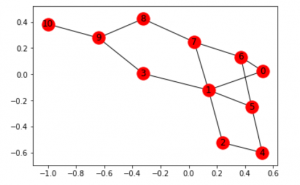Note: The above graph may not look the same on reproduction of the code because the networkx library in python produces a random graph from the given edges.

Step 3: Defining the reward the system for the bot

 `MATRIX_SIZE ``=` `11` `M ``=` `np.matrix(np.ones(shape ``=``(MATRIX_SIZE, MATRIX_SIZE))) ` `M ``*``=` `-``1` ` `  `for` `point ``in` `edges: ` `    ``print``(point) ` `    ``if` `point[``1``] ``=``=` `goal: ` `        ``M[point] ``=` `100` `    ``else``: ` `        ``M[point] ``=` `0` ` `  `    ``if` `point[``0``] ``=``=` `goal: ` `        ``M[point[::``-``1``]] ``=` `100` `    ``else``: ` `        ``M[point[::``-``1``]]``=` `0` `        ``# reverse of point ` ` `  `M[goal, goal]``=` `100` `print``(M) ` `# add goal point round trip `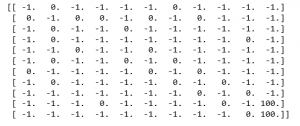Step 4: Defining some utility functions to be used in the training

 `Q ``=` `np.matrix(np.zeros([MATRIX_SIZE, MATRIX_SIZE])) ` ` `  `gamma ``=` `0.75` `# learning parameter ` `initial_state ``=` `1` ` `  `# Determines the available actions for a given state ` `def` `available_actions(state): ` `    ``current_state_row ``=` `M[state, ] ` `    ``available_action ``=` `np.where(current_state_row >``=` `0``)[``1``] ` `    ``return` `available_action ` ` `  `available_action ``=` `available_actions(initial_state) ` ` `  `# Chooses one of the available actions at random ` `def` `sample_next_action(available_actions_range): ` `    ``next_action ``=` `int``(np.random.choice(available_action, ``1``)) ` `    ``return` `next_action ` ` `  ` `  `action ``=` `sample_next_action(available_action) ` ` `  `def` `update(current_state, action, gamma): ` ` `  `  ``max_index ``=` `np.where(Q[action, ] ``=``=` `np.``max``(Q[action, ]))[``1``] ` `  ``if` `max_index.shape[``0``] > ``1``: ` `      ``max_index ``=` `int``(np.random.choice(max_index, size ``=` `1``)) ` `  ``else``: ` `      ``max_index ``=` `int``(max_index) ` `  ``max_value ``=` `Q[action, max_index] ` `  ``Q[current_state, action] ``=` `M[current_state, action] ``+` `gamma ``*` `max_value ` `  ``if` `(np.``max``(Q) > ``0``): ` `    ``return``(np.``sum``(Q ``/` `np.``max``(Q)``*``100``)) ` `  ``else``: ` `    ``return` `(``0``) ` `# Updates the Q-Matrix according to the path chosen ` ` `  `update(initial_state, action, gamma) `

Step 5: Training and evaluating the bot using the Q-Matrix

 `scores ``=` `[] ` `for` `i ``in` `range``(``1000``): ` `    ``current_state ``=` `np.random.randint(``0``, ``int``(Q.shape[``0``])) ` `    ``available_action ``=` `available_actions(current_state) ` `    ``action ``=` `sample_next_action(available_action) ` `    ``score ``=` `update(current_state, action, gamma) ` `    ``scores.append(score) ` ` `  `# print("Trained Q matrix:") ` `# print(Q / np.max(Q)*100) ` `# You can uncomment the above two lines to view the trained Q matrix ` ` `  `# Testing ` `current_state ``=` `0` `steps ``=` `[current_state] ` ` `  `while` `current_state !``=` `10``: ` ` `  `    ``next_step_index ``=` `np.where(Q[current_state, ] ``=``=` `np.``max``(Q[current_state, ]))[``1``] ` `    ``if` `next_step_index.shape[``0``] > ``1``: ` `        ``next_step_index ``=` `int``(np.random.choice(next_step_index, size ``=` `1``)) ` `    ``else``: ` `        ``next_step_index ``=` `int``(next_step_index) ` `    ``steps.append(next_step_index) ` `    ``current_state ``=` `next_step_index ` ` `  `print``(``"Most efficient path:"``) ` `print``(steps) ` ` `  `pl.plot(scores) ` `pl.xlabel(``'No of iterations'``) ` `pl.ylabel(``'Reward gained'``) ` `pl.show() `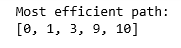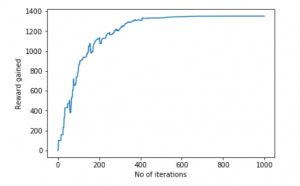Now, Let’s bring this bot to a more realistic setting. Let us imagine that the bot is a detective and is trying to find out the location of a large drug racket. He naturally concludes that the drug sellers will not sell their products in a location which is known to be frequented by the police and the selling locations are near the location of the drug racket. Also, the sellers leave a trace of their products where they sell it and this can help the detective in finding out the required location. We want to train our bot to find the location using these Environmental Clues.

Step 6: Defining and visualizing the new graph with the environmental clues

 `# Defining the locations of the police and the drug traces ` `police ``=` `[``2``, ``4``, ``5``] ` `drug_traces ``=` `[``3``, ``8``, ``9``] ` ` `  `G ``=` `nx.Graph() ` `G.add_edges_from(edges) ` `mapping ``=` `{``0``:``'0 - Detective'``, ``1``:``'1'``, ``2``:``'2 - Police'``, ``3``:``'3 - Drug traces'``, ` `           ``4``:``'4 - Police'``, ``5``:``'5 - Police'``, ``6``:``'6'``, ``7``:``'7'``, ``8``:``'Drug traces'``, ` `           ``9``:``'9 - Drug traces'``, ``10``:``'10 - Drug racket location'``} ` ` `  `H ``=` `nx.relabel_nodes(G, mapping) ` `pos ``=` `nx.spring_layout(H) ` `nx.draw_networkx_nodes(H, pos, node_size ``=``[``200``, ``200``, ``200``, ``200``, ``200``, ``200``, ``200``, ``200``]) ` `nx.draw_networkx_edges(H, pos) ` `nx.draw_networkx_labels(H, pos) ` `pl.show()  `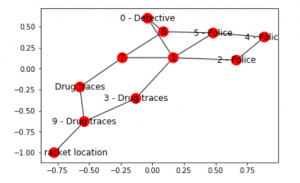Note: The above graph may look a bit different from the previous graph but they, in fact, are the same graphs. This is due to the random placement of nodes by the `networkx `library.

Step 7: Defining some utility functions for the training process

 `Q ``=` `np.matrix(np.zeros([MATRIX_SIZE, MATRIX_SIZE])) ` `env_police ``=` `np.matrix(np.zeros([MATRIX_SIZE, MATRIX_SIZE])) ` `env_drugs ``=` `np.matrix(np.zeros([MATRIX_SIZE, MATRIX_SIZE])) ` `initial_state ``=` `1` ` `  `# Same as above ` `def` `available_actions(state): ` `    ``current_state_row ``=` `M[state, ] ` `    ``av_action ``=` `np.where(current_state_row >``=` `0``)[``1``] ` `    ``return` `av_action ` ` `  `# Same as above ` `def` `sample_next_action(available_actions_range): ` `    ``next_action ``=` `int``(np.random.choice(available_action, ``1``)) ` `    ``return` `next_action ` ` `  `# Exploring the environment ` `def` `collect_environmental_data(action): ` `    ``found ``=` `[] ` `    ``if` `action ``in` `police: ` `        ``found.append(``'p'``) ` `    ``if` `action ``in` `drug_traces: ` `        ``found.append(``'d'``) ` `    ``return` `(found) ` ` `  `  `  `available_action ``=` `available_actions(initial_state) ` `action ``=` `sample_next_action(available_action) ` ` `  `def` `update(current_state, action, gamma): ` `  ``max_index ``=` `np.where(Q[action, ] ``=``=` `np.``max``(Q[action, ]))[``1``] ` `  ``if` `max_index.shape[``0``] > ``1``: ` `      ``max_index ``=` `int``(np.random.choice(max_index, size ``=` `1``)) ` `  ``else``: ` `      ``max_index ``=` `int``(max_index) ` `  ``max_value ``=` `Q[action, max_index] ` `  ``Q[current_state, action] ``=` `M[current_state, action] ``+` `gamma ``*` `max_value ` `  ``environment ``=` `collect_environmental_data(action) ` `  ``if` `'p'` `in` `environment: ` `    ``env_police[current_state, action] ``+``=` `1` `  ``if` `'d'` `in` `environment: ` `    ``env_drugs[current_state, action] ``+``=` `1` `  ``if` `(np.``max``(Q) > ``0``): ` `    ``return``(np.``sum``(Q ``/` `np.``max``(Q)``*``100``)) ` `  ``else``: ` `    ``return` `(``0``) ` `# Same as above ` `update(initial_state, action, gamma) ` ` `  `def` `available_actions_with_env_help(state): ` `    ``current_state_row ``=` `M[state, ] ` `    ``av_action ``=` `np.where(current_state_row >``=` `0``)[``1``] ` ` `  `    ``# if there are multiple routes, dis-favor anything negative ` `    ``env_pos_row ``=` `env_matrix_snap[state, av_action] ` ` `  `    ``if` `(np.``sum``(env_pos_row < ``0``)): ` `        ``# can we remove the negative directions from av_act? ` `        ``temp_av_action ``=` `av_action[np.array(env_pos_row)[``0``]>``=` `0``] ` `        ``if` `len``(temp_av_action) > ``0``: ` `            ``av_action ``=` `temp_av_action ` `    ``return` `av_action ` `# Determines the available actions according to the environment `

Step 8: Visualising the Environmental matrices

 `scores ``=` `[] ` `for` `i ``in` `range``(``1000``): ` `    ``current_state ``=` `np.random.randint(``0``, ``int``(Q.shape[``0``])) ` `    ``available_action ``=` `available_actions(current_state) ` `    ``action ``=` `sample_next_action(available_action) ` `    ``score ``=` `update(current_state, action, gamma) ` ` `  `# print environmental matrices ` `print``(``'Police Found'``) ` `print``(env_police) ` `print``('') ` `print``(``'Drug traces Found'``) ` `print``(env_drugs) `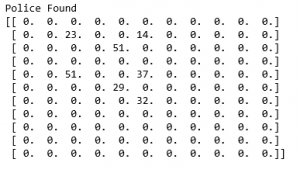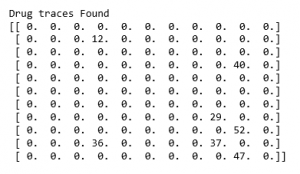Step 9: Training and evaluating the model

 `scores ``=` `[] ` `for` `i ``in` `range``(``1000``): ` `    ``current_state ``=` `np.random.randint(``0``, ``int``(Q.shape[``0``])) ` `    ``available_action ``=` `available_actions_with_env_help(current_state) ` `    ``action ``=` `sample_next_action(available_action) ` `    ``score ``=` `update(current_state, action, gamma) ` `    ``scores.append(score) ` ` `  `pl.plot(scores) ` `pl.xlabel(``'Number of iterations'``) ` `pl.ylabel(``'Reward gained'``) ` `pl.show() `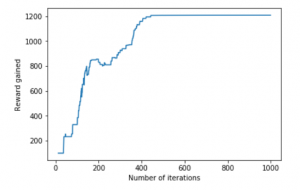The example taken above was a very basic one and many practical examples like Self Driving Cars involve the concept of Game Theory.

My Personal Notes arrow_drop_upCheck out this Author's contributed articles.

If you like GeeksforGeeks and would like to contribute, you can also write an article using contribute.geeksforgeeks.org or mail your article to contribute@geeksforgeeks.org. See your article appearing on the GeeksforGeeks main page and help other Geeks.

Please Improve this article if you find anything incorrect by clicking on the "Improve Article" button below.

Article Tags :
Practice Tags :

2

Please write to us at contribute@geeksforgeeks.org to report any issue with the above content.